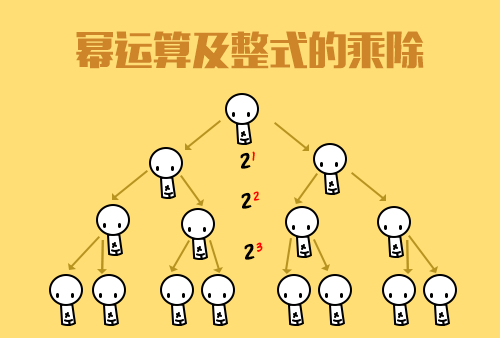| | |

||
APP端下载AndroidiPhone
￥109

|||

|
1766人点赞
758405人已学习
|

3天无理由退款

3天无理由退款：退款将以超级币形式退至您的超级课堂学习账户，便于您重新选购其他课程。恶意退款将被冻结账号。

• 1、一元二次方程的特点，(1).整式方程，(2).只含有一个未知数，(3).未知数的最高次数为$2$
2、 一元二次方程的一般形式，$a$叫做二次项系数，$b$叫做一次项系数，$c$是常数项。只有将方程化为一般形式，才有这些概念
3、 一元二次方程最重要的特征，只需满足二次项系数$a\neq 0$，对$b$和$c$不作限制
4、 代入法：检验数值是否为方程的根，将数值代入方程，验证方程左右两边是否相等
• 1、最简单的直接开平方法，常见的使用情形有三种，$x^{2}=a(a\geq 0)$，$(x-a)^{2}=b(b\geq 0)$，$(x-a)^{2}=k(x-b)^{2}(k＞0)$，遇到这三种情况，直接考虑两边同时开方。但是一定要注意结果正负号的保留
2、 因式分解法求解一元二次方程，分为三步，先把原式化为一般形式，再将等号左边的多项式分解因式。最后，根据乘法原则求出方程的根
• 1、配方法解方程的步骤总结为一首七言绝句：二次系数化为一，常数要往右边移，一次系数一半方，有借有还讲道理
2、 把二次项系数化为$1$后，要配的常数就是一次项系数一半的平方
3、 加上这个常数后，你还一定要减去这个常数，或者在等式的另一边也加上这个常数，这是为了维持等式的恒等
• 1、熟悉万能求根公式$2a$分之$-b$加减根号$b$方减$4ac$的推导过程
2、 将一元二次方程化成一般形式，找出各项系数$a$、$b$、$c$
3、 将$a$、$b$、$c$代入万能求根公式，通过计算求出方程的根
• 1、一元二次方程根的判别式，$\Delta =b^{2}-4ac$。当$\Delta > 2、 0$时，方程有两个不等的根；当$\Delta =0$时，方程有两个相等的根；当$\Delta < 3、 0$时，方程没有根
4、 根据题目告诉你的根的特点，利用判别式可以确定题目中未知参数的取值范围
5、 利用判别式证明一元二次方程根是否存在固定的情况，比如绝对有两个不相等的实数根
6、 根的判别式通常只是解题的第一步，它只能大致判断出根的性质，至于根与系数之间存在的具体关系，这种小纠纷就不在大法官的管辖内了，这时你就要用韦达定理来分析
• 1、韦达定理的基本内容两根之和等于$a$分之$-b$，两根之积等于$a$分之$c$，以及延伸公式$\left | x_{1}-x_{2} \right |$
2、 利用韦达定理表达出两根之间的特殊关系两根的平方和，和两根倒数之和
3、 已知两根之和与两根之积写出原方程$x^{2}+(x_{1}+x_{2})x+x_{1}x_{2}=0$

• 1超级学员233581
• 2超级学员383529
• 3超级学员4
• 47Poll
• 5超级学员603254
• 6超级学员679498
• 7超级学员842570
• 8Yoyo
• 9超级学员865622
• 10及时行乐767546人在学
￥ 330 ￥ 1851306150人在学
￥ 210 ￥ 8756964人在学
￥ 330 ￥ 220755534人在学
￥ 270 ￥ 175

• 0
• 点击分享有好礼
•app端下载关注微信号